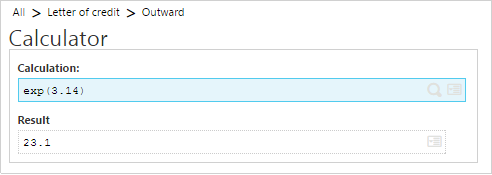# How to find Exponential in Sage Enterprise Management (formerly Sage X3)

By | May 3, 2018

You may come across the situation where you would like to know the exponential of the value. exp function returns its parameter’s exponential.

This function returns the parameter’s exponential (power of e).
exp is the inverse of the In function.
The result type is Double.

Syntax

exp(num_exp)

Element – num_exp

Description – Numeric Expression

Restrictions – -1e80< num_exp <=184

ExampleHope this Helps!